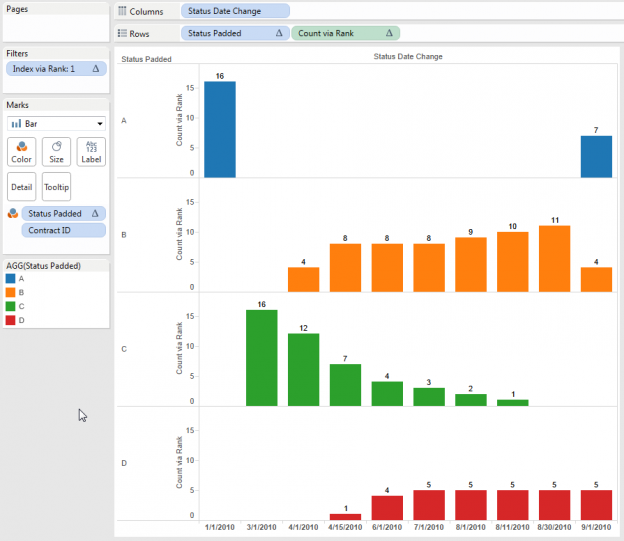# Counting from Nothing – A Double Remix (or, Partitioning via Table Calculations v2)

Over on the Tableau forums Alexander Mou answered a thread on generating a count from sparse data, and the solution he came up with is found in his blog post Dynamic Histogram Over Time. In this post I’m diving into some details of what Alexander did, coming up with a couple of alternative remixes of that solution, and describing a couple of different ways to effectively partition a table calculation via another table calculation. Read on for details!

This is a complicated problem to solve in Tableau because the data doesn’t start out with everything we need. The data has one record per Contract ID and Status Date Change, with a value for the new Status: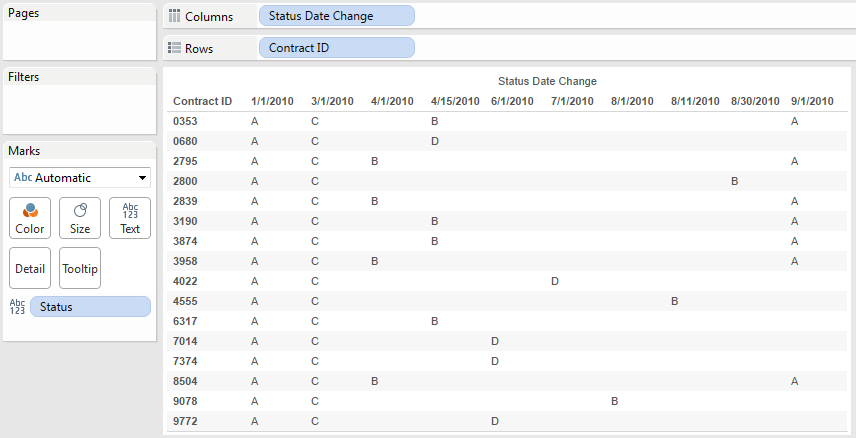Not every status changes on every date, so the data is sparse and if we want to count how many contracts have a given status value on a given date then we need to do some hocus-pocus. A psuedo-code way of putting this in English is “The value of Status at a given date is the most recent value of Status. Then once you have that then count how many contracts have each Status.” So we need to take the above table and make it look like this: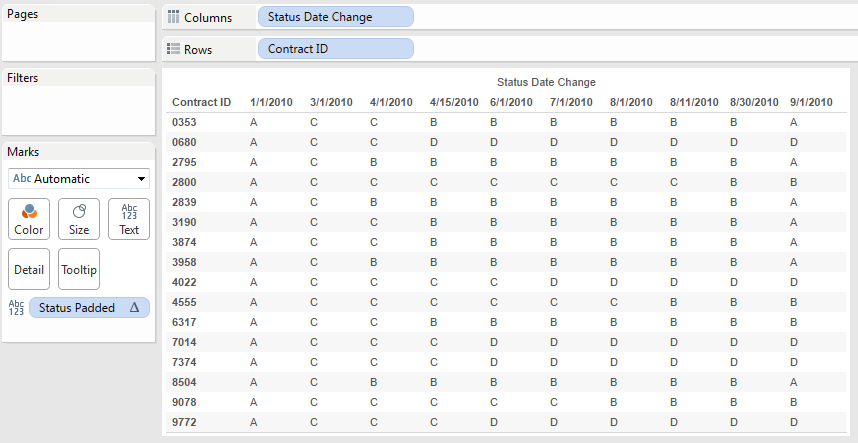In one sense it’s a variation on queues/throughput/census analysis and a way to solve it is via using a custom query to pad out the data, then the count can be done with a simple aggregation. There are SQL examples in the links and posts in that thread.

An alternative solution is based on using Tableau’s data densification, so the padding is done in Tableau – that’s what Alexander used. In another post Alexander had wondered whether SIZE() and/or LOD expressions could be applied, and in response Joshua Milligan explained why not. However, that got me curious as to whether I could come up with something else. I particularly like working through other solutions people have created because building understanding what they did exposes me to alternative ways of thinking and triggers ideas on what I could do.

Here, I propose two alternative remixed solutions that both also use data densification and cut the five calculations down to two (maybe three) in the first remix and three in the second remix.

Both Alexander and I use the same technique to pad out the data, using Tableau’s only iterative function: PREVIOUS_VALUE(). (Or you can do insane things with strings like Noah Salvaterra does.) For a given calculation X, Tableau returns the previous value of X from the prior address in the partition. The argument given for PREVIOUS_VALUE() is only used for the first address in the partition if the first address is Null and sets the data type that is used. For example, if I have a partition with 3 records and use PREVIOUS_VALUE(0)+1, I get the equivalent of an INDEX() or running total: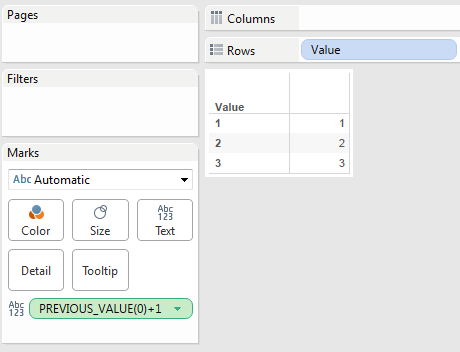In the case of the table, we can pad it out using the following Status Padded calc that has a Compute Using on the Status Date Change:

```IF ISNULL(MIN([Status])) THEN
PREVIOUS_VALUE("")
ELSE
MIN([Status])
END```

The calc takes advantage of Tableau’s order of operations. Normally MIN([Status]) for a non-existent Contract ID–Status Date Change value like 0353–4/1/2010 wouldn’t return anything at all. However, once the data is densified–which will be triggered due to the presence of the PREVIOUS_VALUE()–then MIN([Status]) will return Null, just in time for the ISNULL() function to evaluate to True, at which point the PREVIOUS_VALUE() will return either an empty string or the prior value of Status Padded.  If MIN([Status]) is not Null, then we get a new value of MIN([Status]) to be carried forward.

Now, you might think that you could use LOOKUP([Status Padded],-1) inside the Status Padded calc, but that’s going to give you a “Field [Status Padded] contains a circular reference” error. PREVIOUS_VALUE() is the function to use to get around that.

Here’s the Status Padded function in action:With the Compute Using on Status Date Change (a Date field) and this pill layout, we’re seeing domain completion for all values of Status Date Change & Contract ID based on the pill arrangement and the status values are now fully padded out.

This calc does the same thing as Alexander’s, only with one less function call and this one directly uses MIN([Status]) instead of AVG(1) as the testing value. I tend to like using the actual field because then it can be more apparent what the calc is trying to do.

## Remix #1 – the Brute Force

If Tableau supported partitioning table calculations based on other table calculations, that would be great (please vote for this idea!) and this post would be over just about now because I’d write something like IF FIRST()==0 THEN WINDOW_COUNT(1) END and tell it to address on Status Date Change & Contract ID, partitioning on Status Padded. But we can’t.

I’ve tried describing a workaround for this problem before and didn’t do a very good job, so I’m going to try again. Alexander’s solution does essentially the same thing, only with more calcs than I’m going to use here. Only one more calc is required, the Count via CASE calc has the following formula:

```CASE INDEX()
WHEN 1 THEN WINDOW_SUM(IF [Status Padded] == 'A' THEN 1 ELSE 0 END)
WHEN 2 THEN WINDOW_SUM(IF [Status Padded] == 'B' THEN 1 ELSE 0 END)
WHEN 3 THEN WINDOW_SUM(IF [Status Padded] == 'C' THEN 1 ELSE 0 END)
WHEN 4 THEN WINDOW_SUM(IF [Status Padded] == 'D' THEN 1 ELSE 0 END)
END```

The calc has a nested Compute Using on the Contract ID while the Status Padded retains the Compute Using on Status Date Change.

Count via CASE works by calculating the INDEX() along the Contract ID, and then for each of the first 4 values calculating a WINDOW_SUM() of the number of marks for a given value of Status Padded. Because this is addressing on Contract ID (and therefore partitioning on the Status Date Change), each WINDOW_SUM() is counting up all the marks with a given Status Padded value for each Status Date Change. I call this a “brute force” solution because it’s picking some marks (based on the INDEX()) and then shoving the desired calculated results (the WINDOW_SUM) onto them. It doesn’t actually care what the value of Status Padded is for a given combination of Contract ID–Status Date Change, it’s just using that mark as a placeholder for a value.

If you’ve read this far, you’ve probably had your fare share of mind-expanding moments while learning Tableau. Learning this technique was one of mine, I can still remember the exact workbook that I opened up where Joe Mako used a variation on this technique to get a worksheet with several hundred thousand marks that took several minutes to render down to three values that rendered in a few seconds.

Another factor in thinking this is using “brute force” is that there need to be N WHEN statements for N values of Status in the data, and anytime there’s a new value of Status added to the data the calculation will have to change. Finally, a key limitation of this is that the calculation only works when there are at least as many Contract IDs for a given Status Date Change as there are values of Status. Otherwise the INDEX() will run out of values.

Here’s a view with the Count via CASE calc, we can see that only the first 4 Contract IDs have any values: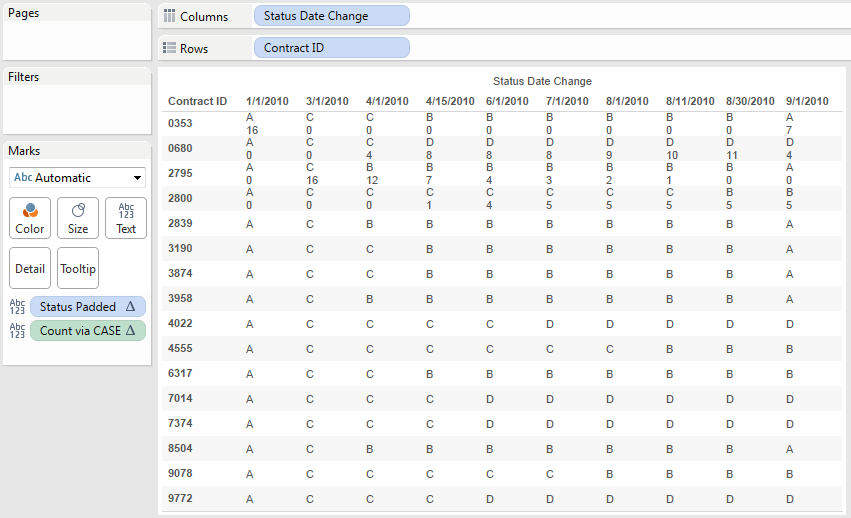Next we can duplicate the worksheet, do a little drag-and-drop to get a bar chart. The one bit that isn’t necessarily obvious is that the Count via CASE on the Filters Shelf is set to filter for Special->non-Null values. This gets rid of the extra 120 marks from the densification and the 120 Null values warning and in views with many more marks can drastically improve performance.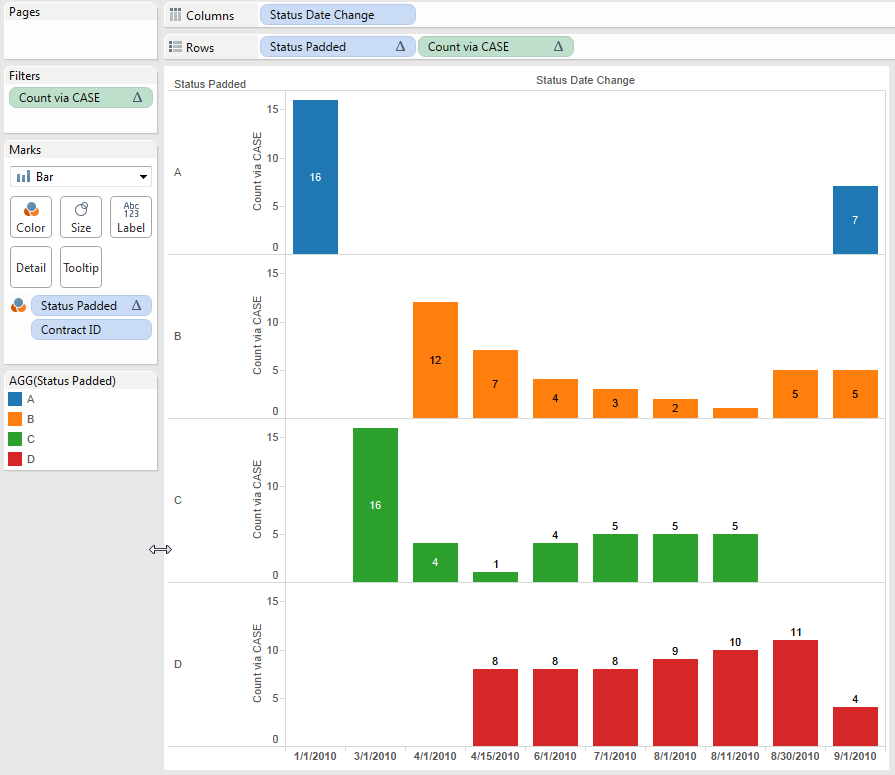Note that this still works because Tableau is still doing densification, only this time it’s switching to date domain completion because the Status Padded calc is addressing on the Status Change Date.

If you want 0s to show up for all the empty bars, you’re going to need another calc. This has to do with how Tableau is laying out the view. I created a “Status for 0s” calc that converts the INDEX values into status values. You could do it via another CASE statement, since the Status values are letters I just used the formula CHAR(INDEX()+64). This returns A for 1, B for 2, etc. With a Compute Using on Contract ID the INDEX() returns the same results as the COUNT() calc and putting the Status for 0s pill on both Rows and Color gets us 0s everywhere: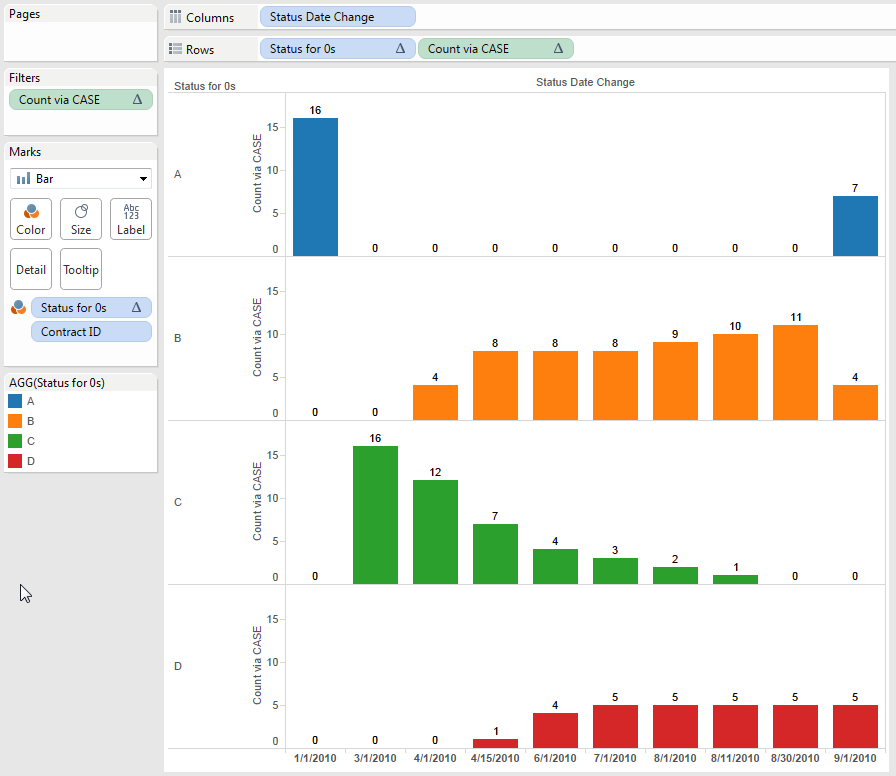So that’s my first remix: one calculation to pad out the data, a second nested calculation to generate the count, and an optional third calculation for getting 0s into the mark labels.

## Remix #2 – Rank to the Rescue

This solution also owes a debt of gratitude to Joe, he took a thread of an idea I’d given up on and extended it into a solution that we can use to count an arbitrary number of things inside a partition. It takes advantage of the assorted RANK functions to do two things:

1. Count up the number of elements of each Status Padded in a partition (the Status Date Change) using the formula RANK_MODIFIED([Status Padded]) – RANK([Status Padded]) +1 with a nested Compute Using on the Contract ID. This returns an accurate count for each value of Status ID for every (padded) Contract ID–Status Change ID combination: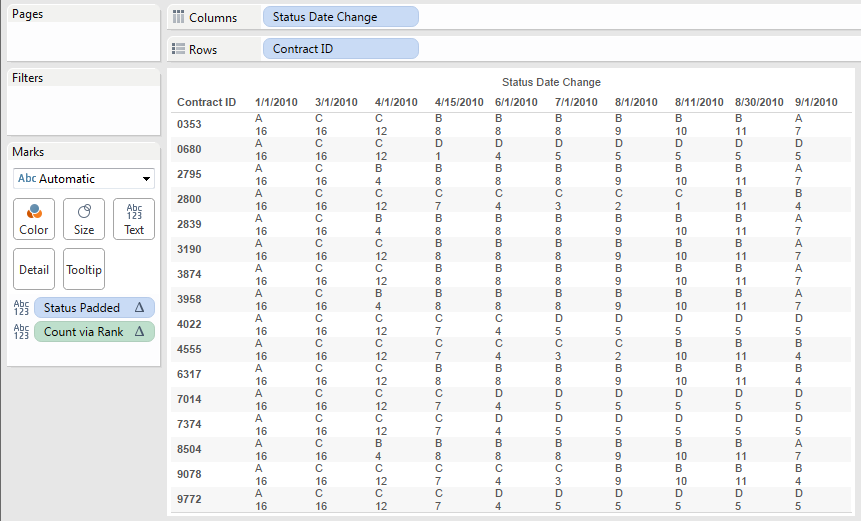The calc works by getting the RANK_MODIFIED() that puts ties lower, so 5,5,3 is ranked as 2,2,3 and then subtracting the RANK() that puts ties higher so 5,5,3 is ranked as 1,1,3 leads to 1,1,0. Adding back 1 to each because the RANK calcs are 1-based leads to 2,2,1, which is exactly the count for each value.

However, returning the count for each value causes a problem if we just duplicate the view and start moving pills around. We only need 40 values for the Count: one for each combination of Status Padded and Status Date Change. Since this view has 160 values, we’ll end up with duplicate marks. It’s possible to build a view and use Analysis->Stack Marks->Off, but I don’t like doing that because it’s making Tableau draw more marks than necessary plus a user could click+drag to select a mark and end up getting the whole stack, which could lead to some erroneous conclusions.

An INDEX() or IF FIRST()==0 calc here to get the marks won’t work because the data isn’t sorted in any way that would be meaningful. So, we need to resort to rank again…

2. The next use of RANK calcs is to generate an index for each value of Status Padded where the 1st mark for a given value has a 1, the 2nd mark a value of 2, and so on. The Index via Rank calc does this using the formula RANK_UNIQUE([Status Padded]) – RANK([Status Padded]) + 1. For a set of values 5,5,3,2,2 RANK_UNIQUE() will return 1,2,3,4,5. For the values 5,5,3,2 RANK() returns 1,1,3,4,4. [1,2,3,4,5] – [1,1,3,4,4] = [0,1,0,0,1] Adding 1 to that (again because the RANK calcs are 1-based) leads to [1,2,1,1,2], so we’ve generated an index on each of the original values. Finally if we filter for just the 1’s we’ll end up with the first of each of the distinct values: 5,3.2.Here’s the Index via Rank calc in the view. With Index via Rank as a discrete on Rows, we can see how each Status Padded gets a different Rank value, and set of values for Index via Rank = 1 is complete.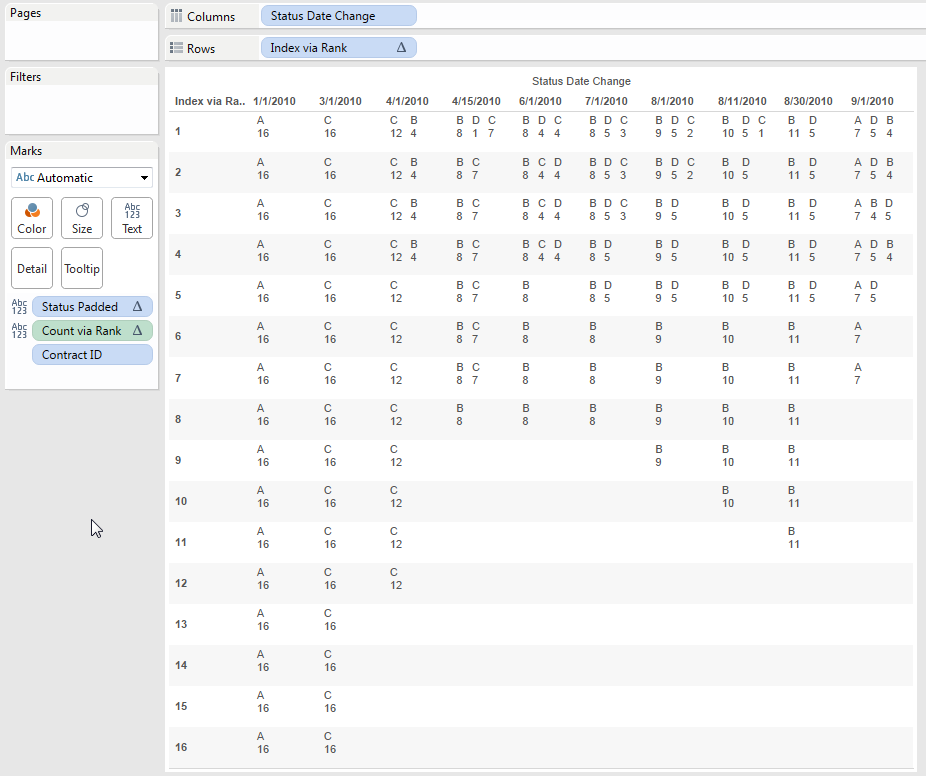Now if we just filter for the 1’s we can end up with just enough marks to draw a set of bars: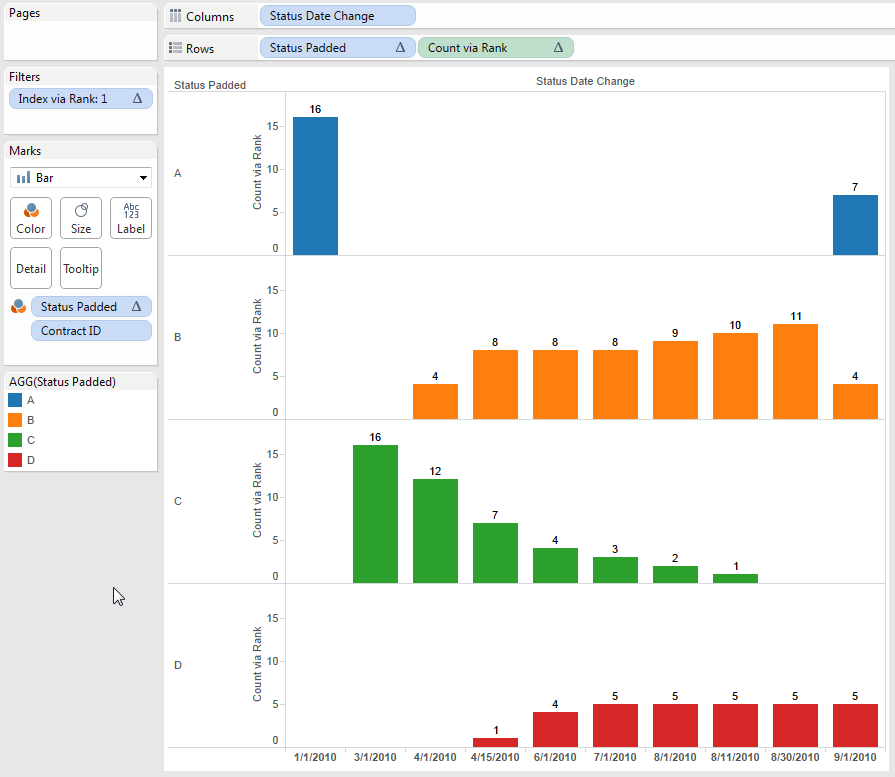A big advantage of using these two rank formulas to generate the count is that it doesn’t require the hard-coding of the brute force solution. However, due to the way they work we don’t get to see 0s if we want to, because the rank formulas are directly displaying the results within each Status Date Change whereas the brute force solution uses an indirection.

## Conclusion

So, those are two ways you can effectively partition table calculations via another table calculation. Hopefully this second time around is a bit more understandable than the first!  From what I’ve seen on the forums since v9 came out I’m expecting that these techniques will be less necessary because there are so many cases where a FIXED LOD expression can provide the computational result that can be used as a regular dimension to partition the view.

Here’s the Tableau Public workbook used in this post: tracking status remix

## 3 thoughts on “Counting from Nothing – A Double Remix (or, Partitioning via Table Calculations v2)”

1.Alexander Mou

Great work! Learnt a few tricks again. Mind boggling though. Btw, the padding formula was created by Nicolas Steinbach who posted the original question.

2.graskaas2014

Wow great post! You mention this work around tactic would not be needed with v9 and FIXED level of detail calcs. Would you like to elaborate on how this could be done with level of detail calc?
Would be much appreciated and thanks in advance.

1.Jonathan Drummey Post author